# Buoyant Forces

The behavior of an object submerged in a fluid is governed by Archimedes' Principle. Archimedes (a greek mathematician) determined that a body which is completely or partially submerged in a fluid experiences an upward force called the Buoyant Force, B , which is equal in magnitude to the weight of the fluid displaced by the object. This principle can be used to explain why ships, loaded with millions of kilograms of cargo, are able to float.

To prove Archimedes' Principle, consider an object emmersed in a fluid, as shown in the Fig.9.7.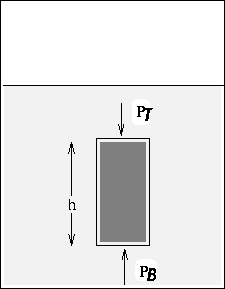The buoyant force is due to the difference between the pressure at the bottom of the object pushing up on it, and the pressure at the top pushing down. Thus:

 B = (PB - PT)A (14)

where we assume that the top and bottom of the object have the same area A .

Previously, we showed that the pressure at the bottom of the cube is greater than that at the top by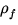gh , whereis the density of the fluid and h is the height of the object. Substituting this into the above equation gives:

B =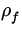ghA =Vg = mfg = wf

where V = hA is the volume of the displaced fluid and wf = mfg =Vg is the weight of the displaced fluid. This proves Archimedes' principle:

B = wf

This result holds true for an object of any size, density or shape which is totally submerged.

Note:

• If an object is totally submerged, then Vf = Vo , where Vo is the total volume of the object. The net upward force on a completely submerged object is therefore:

B - wo = (-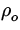)Vog

where we have used the fact that the weight of the object wo =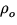gVo . Thus, whether a submerged object sinks or rises to the surface is determined by the relative magnitude ofand. If>, the object rises, if<, then the object sinks.
• If an object is floating on the surface (neither sinking nor rising further) then the volume that is below the surface can be determined by requiring the net force on the object to be zero:

B - wo =gVf - wo = 0

where Vf is the volume of the object that is submerged (which equals the volume of displaced fluid) and wo is the total weight of the object. If the object has volume Vo and uniform density, then the fraction of the volume below the surface is:=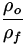.Next: Fluids in Motion Up: Solids and Fluids Previous: Pressure Measurements